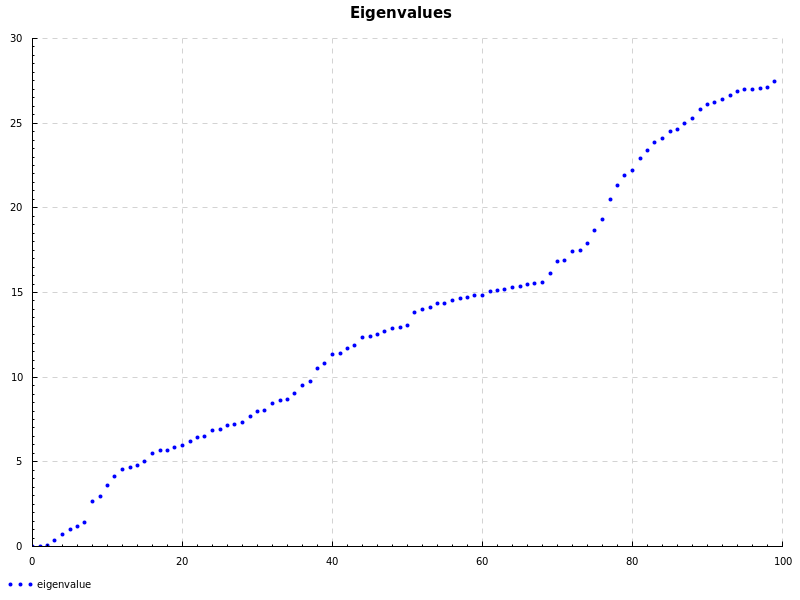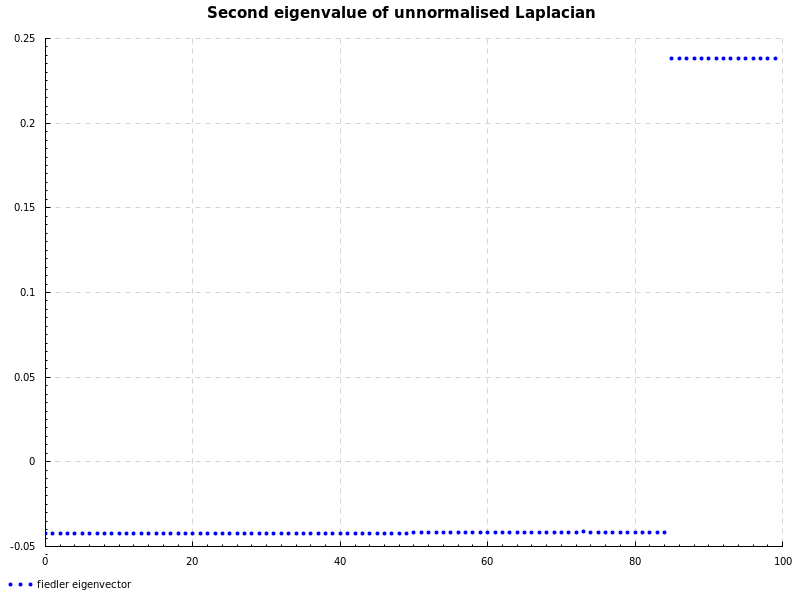I was reading some notes from QUT about using spectral graph theory to partition a graph using eigenvalues of unnormalised Laplacian. Their Matlab code looks like this:

% Form W = Gaussian distribution based on distances
W = zeros(100, 100);
D = zeros(100, 100);

sigma = 2;
N = 100;
for i = 1:N
for j = 1:N
if (j ~= i)
% Calculate the distance between two points
dist = norm([A(i, 1) - A(j, 1); A(i, 2) - A(j, 2)]);
expp = exp(-dist^2 / (2 * sigma^2));
% Add the weights to the matrix
W(i, j) = expp;
% Add up the row sum as we go
D(i, i) = D(i, i) + expp;
end
end
end

L = D - W;

Find the eigenpairs of L

[vec, val] = eig(L, 'vector');
% Ensure the eigenvalues and eigenvectors are sorted in ascending order
[val,ind] = sort(val);
vec = vec(:, ind);

% Plot with clusters identified by marker
% v2
figure;
hold on;
for i = 1:N
plot(i, vec(i, 2), ['k' plot_syms{i}]);
end


Personally, this gives me nightmares from undergrad numerical methods classes. So here’s how to do it in Haskell. Full source (including cabal config) for this post is here.

To create the W matrix we use buildMatrix:

The D matrix is a diagonal matrix with each element being the sum of a row of W, which is nicely expressible by composing a few functions:

The L matrix is real and symmetric so we use eigSH which provides the eigenvalues in descending order.

To finish up, the Fiedler eigenvector corresponds to the second smallest eigenvalue:

To plot the eigenvalues and eigenvector we use the Chart library which uses the cairo backend.